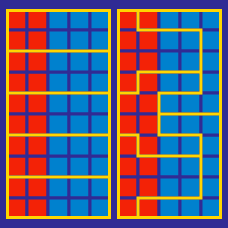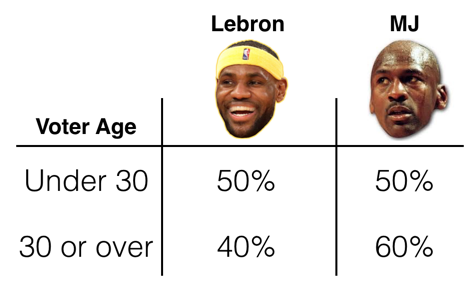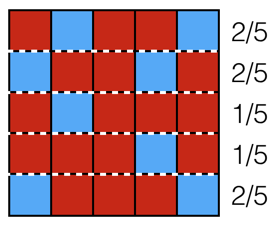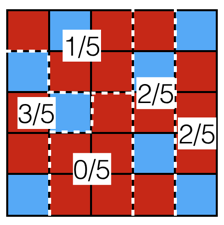Logic

# Math of Voting WarmupBased on the results above, from a survey of basketball fans about the best player of all time, who did more fans vote for? (Note: Assume the number of voters under 30 was the same as the number of voters 30 or over.)

Three states are being allocated 62 seats in a legislative body. State A has 17% of the population, State B has 37%, and State C has 46%.

Applying these percentages to the 62 seats and rounding to the nearest integer means State A gets 11 seats, State B gets 23 seats, and State C gets 29 seats.

What’s wrong with this approach?In the map above, the Blue party wins none of the five voting regions. If voting regions must contain 5 connected squares, what is the most voting regions the Blue party could win?

For example, if the voting regions were drawn like this, they would win 1:A political score $P$ is a number between 0 and 100 where $P=0$ represents the extreme left-wing and $P=100$ represents the extreme right-wing. A candidate's positions are also assigned a score, and each voter will vote for whichever candidate has positions with a score closest to their own.

In a two-candidate race where each candidate wants to get as many votes as possible, what position(s) will they take?

Note: In the case where two candidates are equidistant from a voter, assume the voter decides randomly.

There are four candidates in the beginning of an election: 1, 2, 3, and 4. Given the preferences of the four voters below, the only head-to-head race that will not end in a tie is between candidates numbered $A$ and $B.$ What is $A \times B?$

\begin{aligned} \text{Alexa:} \ 1 &> 2 > 3 > 4 \\ \text{Benny:} \ 2 &> 1> 4> 3 \\ \text{Celina:} \ 3 &> 4 > 2 > 1 \\ \text{Dakota:} \ 4&>1>3>2 \\ \end{aligned}

×